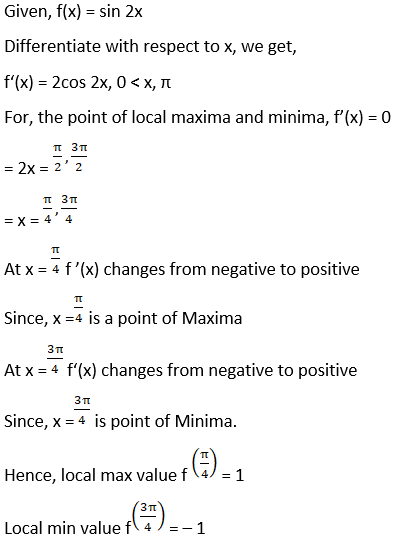# RD Sharma Solutions For Class 12 Maths Exercise 18.2 Chapter 18 Maxima and Minima

RD Sharma Solutions for Class 12 Maths Exercise 18.2 Chapter 18 Maxima and Minima are provided here. Experts at BYJU’S suggest students practice the solutions many number of times to yield good results in their exams. Our expert team has designed the solutions for RD Sharma Solutions for Class 12 Maths Chapter 18 to help students prepare for their exams with ease.

The second exercise of Chapter 18 Maxima and Minima explains local maxima and local minima of a given function. Here PDF of this exercise can be downloaded from the given links. Some of the important topics of this exercise are listed below.

• Local maxima
• Local minima
• First derivative test for local maxima and minima

## Download the PDF of RD Sharma Solutions For Class 12 Chapter 18 – Maxima and Minima Exercise 18.2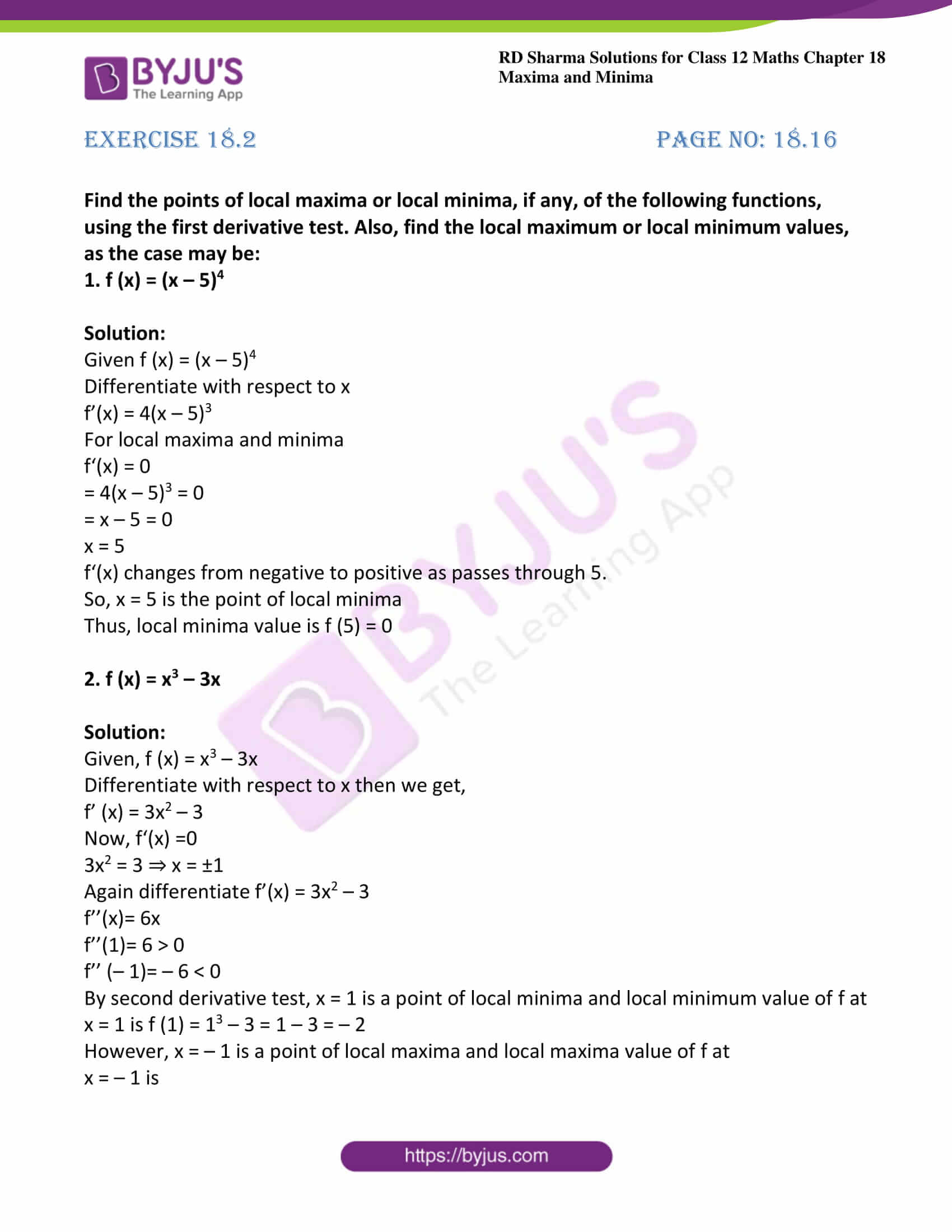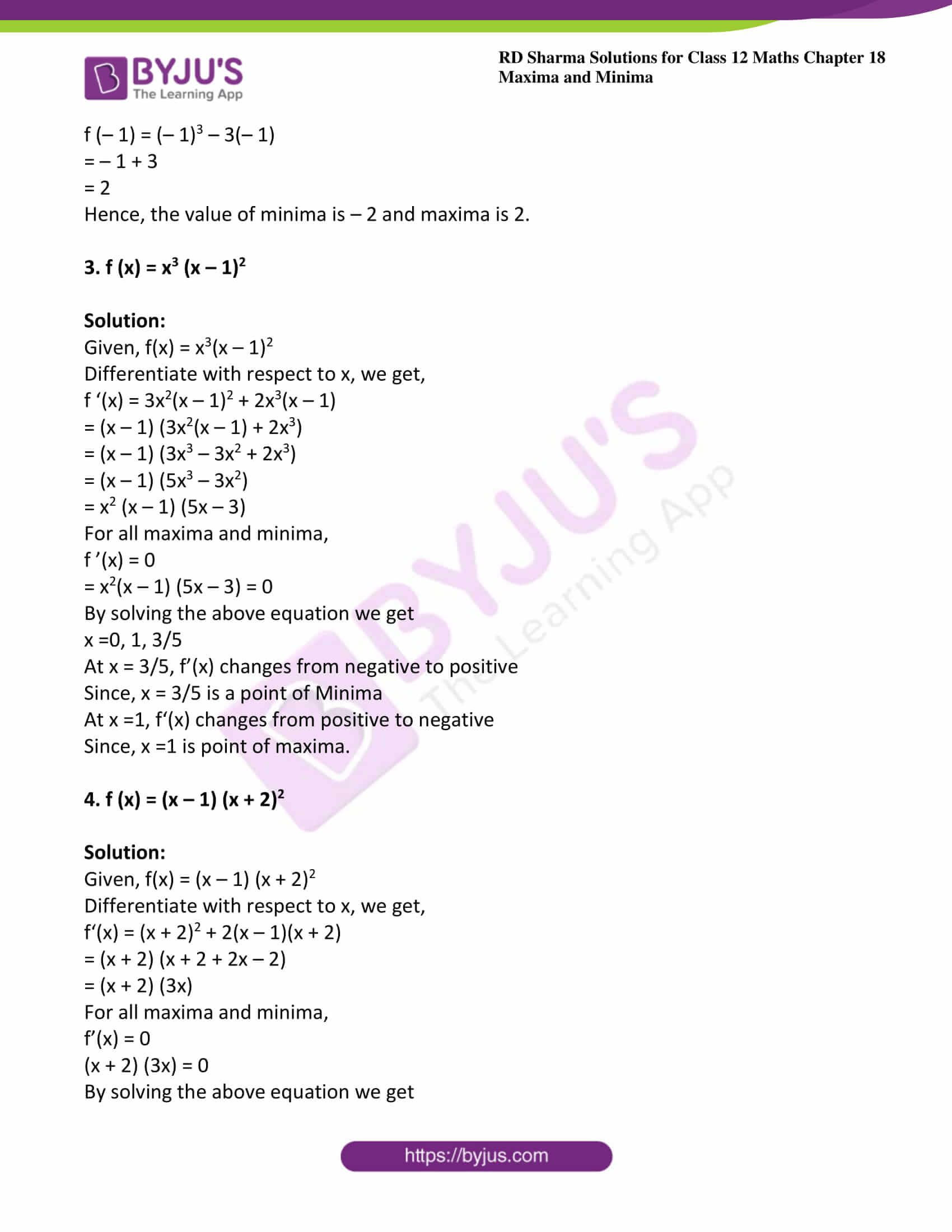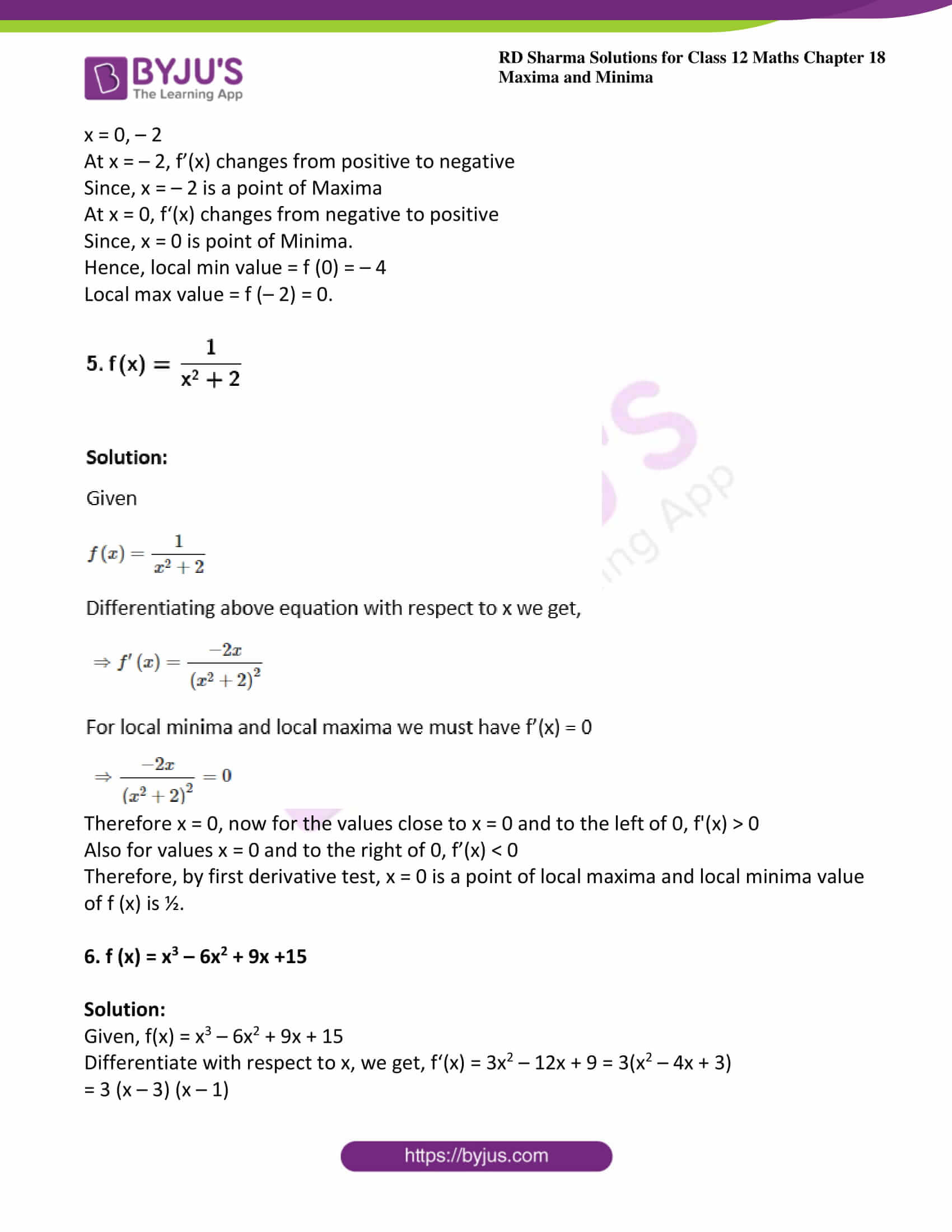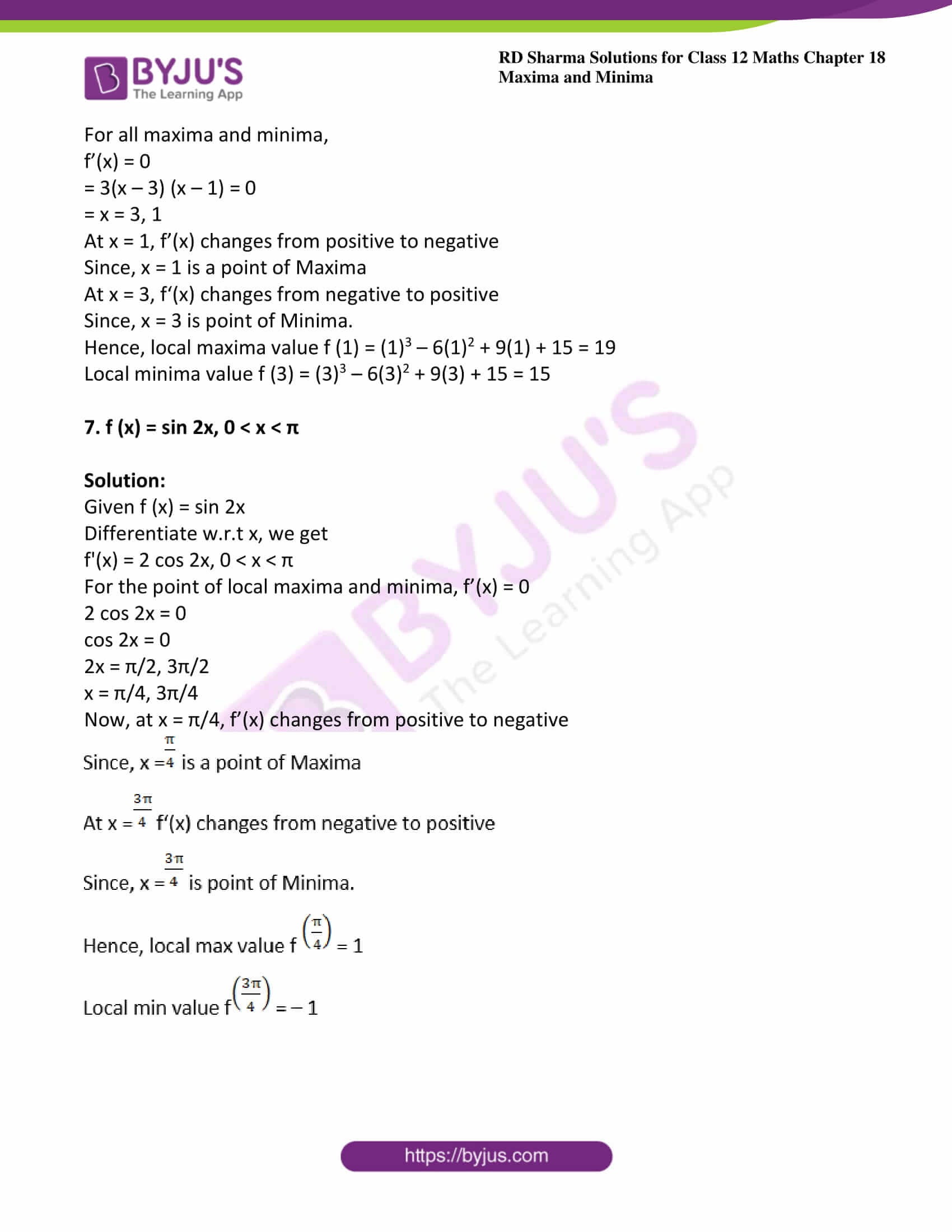### Access other exercises of RD Sharma Solutions For Class 12 Chapter 18 – Maxima and Minima

Exercise 18.1 Solutions

Exercise 18.3 Solutions

Exercise 18.4 Solutions

Exercise 18.5 Solutions

### Exercise 18.2 Page No: 18.16

Find the points of local maxima or local minima, if any, of the following functions, using the first derivative test. Also, find the local maximum or local minimum values, as the case may be:

1. f (x) = (x – 5)4

Solution:

Given f (x) = (x – 5)4

Differentiate with respect to x

f’(x) = 4(x – 5)3

For local maxima and minima

f‘(x) = 0

= 4(x – 5)3 = 0

= x – 5 = 0

x = 5

f‘(x) changes from negative to positive as passes through 5.

So, x = 5 is the point of local minima

Thus, local minima value is f (5) = 0

2. f (x) = x3 – 3x

Solution:

Given, f (x) = x3 – 3x

Differentiate with respect to x then we get,

f’ (x) = 3x2 – 3

Now, f‘(x) =0

3x2 = 3 ⇒ x = ±1

Again differentiate f’(x) = 3x2 – 3

f’’(x)= 6x

f’’(1)= 6 > 0

f’’ (– 1)= – 6 < 0

By second derivative test, x = 1 is a point of local minima and local minimum value of f at

x = 1 is f (1) = 13 – 3 = 1 – 3 = – 2

However, x = – 1 is a point of local maxima and local maxima value of f at

x = – 1 is

f (– 1) = (– 1)3 – 3(– 1)

= – 1 + 3

= 2

Hence, the value of minima is – 2 and maxima is 2.

3. f (x) = x3 (x – 1)2

Solution:

Given, f(x) = x3(x – 1)2

Differentiate with respect to x, we get,

f ‘(x) = 3x2(x – 1)2 + 2x3(x – 1)

= (x – 1) (3x2(x – 1) + 2x3)

= (x – 1) (3x3 – 3x2 + 2x3)

= (x – 1) (5x3 – 3x2)

= x2 (x – 1) (5x – 3)

For all maxima and minima,

f ’(x) = 0

= x2(x – 1) (5x – 3) = 0

By solving the above equation we get

x =0, 1, 3/5

At x = 3/5, f’(x) changes from negative to positive

Since, x = 3/5 is a point of Minima

At x =1, f‘(x) changes from positive to negative

Since, x =1 is point of maxima.

4. f (x) = (x – 1) (x + 2)2

Solution:

Given, f(x) = (x – 1) (x + 2)2

Differentiate with respect to x, we get,

f‘(x) = (x + 2)2 + 2(x – 1)(x + 2)

= (x + 2) (x + 2 + 2x – 2)

= (x + 2) (3x)

For all maxima and minima,

f’(x) = 0

(x + 2) (3x) = 0

By solving the above equation we get

x = 0, – 2

At x = – 2, f’(x) changes from positive to negative

Since, x = – 2 is a point of Maxima

At x = 0, f‘(x) changes from negative to positive

Since, x = 0 is point of Minima.

Hence, local min value = f (0) = – 4

Local max value = f (– 2) = 0.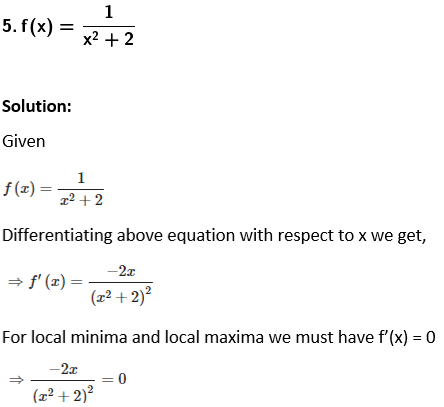Therefore x = 0, now for the values close to x = 0 and to the left of 0, f'(x) > 0

Also for values x = 0 and to the right of 0, f’(x) < 0

Therefore, by first derivative test, x = 0 is a point of local maxima and local minima value of f (x) is ½.

6. f (x) = x3 – 6x2 + 9x +15

Solution:

Given, f(x) = x3 – 6x2 + 9x + 15

Differentiate with respect to x, we get, f‘(x) = 3x2 – 12x + 9 = 3(x2 – 4x + 3)

= 3 (x – 3) (x – 1)

For all maxima and minima,

f’(x) = 0

= 3(x – 3) (x – 1) = 0

= x = 3, 1

At x = 1, f’(x) changes from positive to negative

Since, x = 1 is a point of Maxima

At x = 3, f‘(x) changes from negative to positive

Since, x = 3 is point of Minima.

Hence, local maxima value f (1) = (1)3 – 6(1)2 + 9(1) + 15 = 19

Local minima value f (3) = (3)3 – 6(3)2 + 9(3) + 15 = 15

7. f (x) = sin 2x, 0 < x < π

Solution:

Given f (x) = sin 2x

Differentiate w.r.t x, we get

f'(x) = 2 cos 2x, 0 < x < π

For the point of local maxima and minima, f’(x) = 0

2 cos 2x = 0

cos 2x = 0

2x = π/2, 3π/2

x = π/4, 3π/4

Now, at x = π/4, f’(x) changes from positive to negative